# End-to-End DVB-S2 Simulation with RF Impairments and Corrections

This example shows how to measure the bit error rate (BER) and packet error rate (PER) of a single stream Digital Video Broadcasting Satellite Second Generation (DVB-S2) link that has constant coding and modulation. The example describes the symbol timing and carrier synchronization strategies in detail, emphasizing how to estimate the RF front-end impairments under heavy noise conditions. The single stream signal adds RF front-end impairments and then passes the waveform through an additive white Gaussian noise (AWGN) channel.

### Introduction

DVB-S2 receivers are subjected to large carrier frequency errors in the order of 20% of the input symbol rate and substantial phase noise. The use of powerful forward error correction (FEC) mechanisms, such as Bose–Chaudhuri–Hocquenghem (BCH) and low density parity check (LDPC) codes, caused the DVB-S2 system to work at very low energy per symbol to noise power spectral density ratio (${\mathit{E}}_{\mathit{s}}/{\mathit{N}}_{\mathit{o}}$) values, close to the Shannon limit.

ETSI EN 302 307-1 Section 6 Table 13  summarizes the Quasi-Error-Free (QEF) performance requirement over an AWGN channel for different modulation schemes and code rates. The operating ${\mathit{E}}_{\mathit{s}}/{\mathit{N}}_{\mathit{o}}$ range for different transmission modes can be considered as +2 or -2 dB from the ${\mathit{E}}_{\mathit{s}}/{\mathit{N}}_{\mathit{o}}$ point where QEF performance is observed. Because the operating ${\mathit{E}}_{\mathit{s}}/{\mathit{N}}_{\mathit{o}}$ range is low, carrier and symbol timing synchronization strategies are challenging design problems.

This diagram summarizes the example workflow.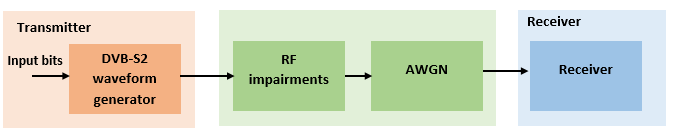#### Main Processing Loop

The example processes 25 physical layer (PL) frames of data with the ${\mathit{E}}_{\mathit{s}}/{\mathit{N}}_{\mathit{o}}$ set to 20 dB, and then computes the BER and PER. Carrier frequency offset, sampling clock offset, and phase noise impairments are applied to the modulated signal, and AWGN is added to the signal.

At the receiver, after matched filtering, timing and carrier recovery operations are run to recover the transmitted data. To extract PL frames, the distorted waveform is processed through various timing and carrier recovery strategies to extract PL frames. The carrier recovery algorithms are pilot-aided. To decode the data frames, the physical layer transmission parameters such as modulation scheme, code rate, and FEC frame type, are recovered from the PL header. To regenerate the input bit stream, the baseband (BB) header is decoded.

Because the DVB-S2 standard supports packetized and continuous modes of transmission, the BB frame can be either a concatenation of user packets or a stream of bits. The BB header is recovered to determine the mode of transmission. If the BB frame is a concatenation of user packets, the packet cyclic redundancy check (CRC) status of each packet is returned along with the decoded bits, and then the PER and BER are measured.

These block diagrams show the synchronization and input bit recovery workflows.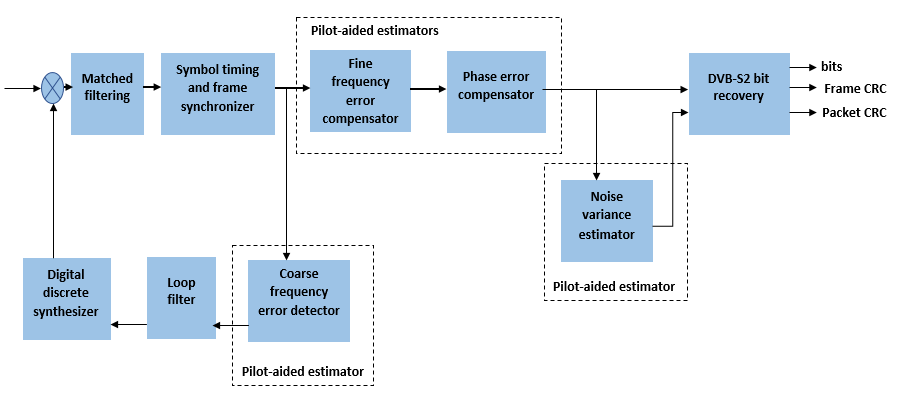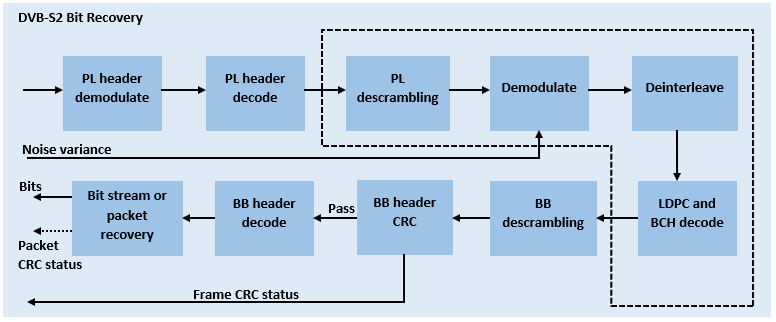This example loads a MAT-file with DVB-S2 LDPC parity matrices. If the MAT-file is not available on the MATLAB® path, use these commands to download and unzip the MAT-file.

```if ~exist('dvbs2xLDPCParityMatrices.mat','file') if ~exist('s2xLDPCParityMatrices.zip','file') url = 'https://ssd.mathworks.com/supportfiles/spc/satcom/DVB/s2xLDPCParityMatrices.zip'; websave('s2xLDPCParityMatrices.zip',url); unzip('s2xLDPCParityMatrices.zip'); end addpath('s2xLDPCParityMatrices'); end```

### DVB-S2 Configuration in Pilot-Aided Mode

Specify the `cfgDVBS2` structure to define DVB-S2 transmission configuration parameters. The `ScalingMethod` property applies when `MODCOD` is in the range [18, 28] (that is, when the modulation scheme is APSK only). `UPL` property is applicable when you set the `StreamFormat` to "`GS`".

```cfgDVBS2.StreamFormat = "TS"; cfgDVBS2.FECFrame = "normal"; cfgDVBS2.MODCOD = 18; % 16APSK 2/3 cfgDVBS2.DFL = 42960; cfgDVBS2.ScalingMethod = "Unit average power"; cfgDVBS2.RolloffFactor = 0.35; cfgDVBS2.HasPilots = true; cfgDVBS2.SamplesPerSymbol = 2```
```cfgDVBS2 = struct with fields: StreamFormat: "TS" FECFrame: "normal" MODCOD: 18 DFL: 42960 ScalingMethod: "Unit average power" RolloffFactor: 0.3500 HasPilots: 1 SamplesPerSymbol: 2 ```

### Simulation Parameters

The DVB-S2 standard supports flexible channel bandwidths. Use a typical channel bandwidth such as 36 MHz. The channel bandwidth can be varied. The coarse frequency synchronization algorithm implemented in this example can track carrier frequency offsets up to 20% of the input symbol rate. The symbol rate is calculated as B/(1+R), where B is the channel bandwidth, and R is the transmit filter roll-off factor. The algorithms implemented in this example can correct the sampling clock offset up to 10 ppm.

```simParams.sps = cfgDVBS2.SamplesPerSymbol; % Samples per symbol simParams.numFrames = 25; % Number of frames to be processed simParams.chanBW = 36e6; % Channel bandwidth in Hertz simParams.cfo = 3e6; % Carrier frequency offset in Hertz simParams.sco = 5; % Sampling clock offset in parts % per million simParams.phNoiseLevel =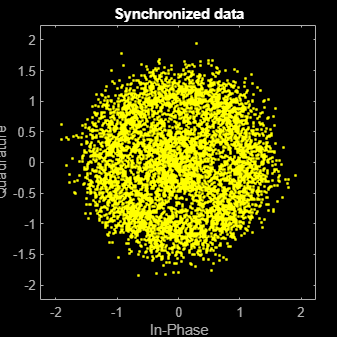"Low"; % Phase noise level provided as % 'Low', 'Medium', or 'High' simParams.EsNodB = 20; % Energy per symbol to noise ratio % in decibels```

This table defines the phase noise mask (dBc/Hz) used to generate the phase noise applied to the transmitted signal.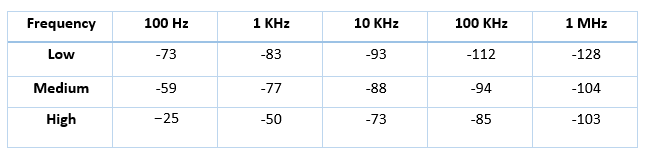### Generate DVB-S2 Waveform Distorted with RF Impairments

To create a DVB-S2 waveform, use the `HelperDVBS2RxInputGenerate` helper function with the `simParams` and `cfgDVBS2` structures as inputs. The function returns the data signal, transmitted and received waveforms, and a receiver processing structure. The received waveform is impaired with carrier frequency, timing phase offsets, and phase noise and then passed through an AWGN channel. The receiver processing parameters structure, `rxParams`, includes the reference pilot fields, pilot indices, counters, and buffers. Plot the constellation of the received symbols and the spectrum of the transmitted and received waveforms.

```[data,txOut,rxIn,rxParams] = HelperDVBS2RxInputGenerate(cfgDVBS2,simParams); % Received signal constellation plot rxConst = comm.ConstellationDiagram('Title','Received data', ... 'XLimits',[-1 1],'YLimits',[-1 1], ... 'ShowReferenceConstellation',false, ... 'SamplesPerSymbol',simParams.sps); rxConst(rxIn(1:length(txOut)))```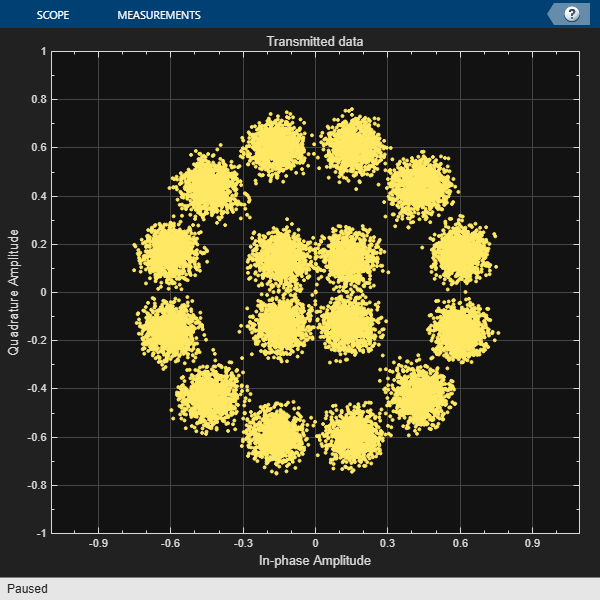```% Transmitted and received signal spectrum visualization Rsymb = simParams.chanBW/(1 + cfgDVBS2.RolloffFactor); Fsamp = Rsymb*simParams.sps; specAn = dsp.SpectrumAnalyzer('SampleRate',Fsamp, ... 'ChannelNames',{'Transmitted waveform','Received waveform'}, ... 'ShowLegend',true); specAn([txOut, rxIn(1:length(txOut))]);```At the receiver, symbol timing synchronization is performed on the received data and is then followed by frame synchronization. The receiver algorithms include coarse and fine frequency impairment correction algorithms. The carrier frequency estimation algorithm can track carrier frequency offsets up to 20% of the input symbol rate. The coarse frequency estimation, implemented as a frequency locked loop (FLL), reduces the frequency offset to a level that the fine frequency estimator can track. The preferred loop bandwidth for symbol timing and coarse frequency compensation depends on the ${\mathit{E}}_{\mathit{s}}/{\mathit{N}}_{\mathit{o}}$ setting.

A block of 36 pilots is repeated every 1476 symbols. The coarse frequency error estimation uses 34 of the 36 pilot symbols. The ratio of used pilots per block (34) and pilot periodicity (1476) is 0.023. Using the 0.023 value as a scaling factor for the coarse frequency synchronizer loop bandwidth is preferred.

When you decrease the ${\mathit{E}}_{\mathit{s}}/{\mathit{N}}_{\mathit{o}}$, you can reduce the loop bandwidth to filter out more noise during acquisition. The number of frames required for the symbol synchronizer and coarse FLL to converge depends on the loop bandwidth setting.

The frame synchronization uses the PL header. Because the carrier synchronization is data-aided, the frame synchronization must detect the start of frame accurately. ${\mathit{E}}_{\mathit{s}}/{\mathit{N}}_{\mathit{o}}$ plays a crucial role in determining the accuracy of the frame synchronization. When QPSK modulated frames are being recovered at ${\mathit{E}}_{\mathit{s}}/{\mathit{N}}_{\mathit{o}}$values below 3 dB, the frame synchronization must be performed on multiple frames for accurate detection.

The fine frequency estimation can track carrier frequency offsets up to 4% of the input symbol rate. The fine frequency estimation must process multiple pilot blocks for the residual carrier frequency offset to be reduced to levels acceptable for the phase estimation algorithm. The phase estimation algorithm can handle residual carrier frequency errors less than 0.02% of the input symbol rate. Fine phase compensation is only required for APSK modulation schemes in the presence of significant phase noise.

These settings are assigned in the `rxParams` structure for synchronization processing. For details on how to set these parameters for low ${\mathit{E}}_{\mathit{s}}/{\mathit{N}}_{\mathit{o}}$ values, see the Further Exploration section.

```rxParams.carrSyncLoopBW = 1e-2*0.023; % Coarse frequency estimator loop bandwidth % normalized by symbol rate rxParams.symbSyncLoopBW = 8e-3; % Symbol timing synchronizer loop bandwidth % normalized by symbol rate rxParams.symbSyncLock = 6; % Number of frames required for symbol % timing error convergence rxParams.frameSyncLock = 1; % Number of frames required for frame % synchronization rxParams.coarseFreqLock = 3; % Number of frames required for coarse % frequency acquisition rxParams.fineFreqLock = 6; % Number of frames required for fine % frequency estimation rxParams.hasFinePhaseCompensation = false; % Flag to indicate whether fine phase % compensation is used rxParams.finePhaseSyncLoopBW = 3.5e-4; % Fine phase compensation loop bandwidth % normalized by symbol rate % Total frames taken for symbol timing and coarse frequency lock to happen rxParams.initialTimeFreqSync = rxParams.symbSyncLock + rxParams.frameSyncLock + ... rxParams.coarseFreqLock; % Total frames used for overall synchronization rxParams.totalSyncFrames = rxParams.initialTimeFreqSync + rxParams.fineFreqLock; % Create time frequency synchronization System object by using % HelperDVBS2TimeFreqSynchronizer helper object timeFreqSync = HelperDVBS2TimeFreqSynchronizer( ... 'CarrSyncLoopBW',rxParams.carrSyncLoopBW, ... 'SymbSyncLoopBW',rxParams.symbSyncLoopBW, ... 'SamplesPerSymbol',simParams.sps, ... 'DataFrameSize',rxParams.xFecFrameSize, ... 'SymbSyncTransitFrames',rxParams.symbSyncLock, ... 'FrameSyncAveragingFrames',rxParams.frameSyncLock); % Create fine phase compensation System object by using % HelperDVBS2FinePhaseCompensator helper object. Fine phase % compensation is only required for 16 and 32 APSK modulated frames if cfgDVBS2.MODCOD >= 18 && rxParams.hasFinePhaseCompensation finePhaseSync = HelperDVBS2FinePhaseCompensator( ... 'DataFrameSize',rxParams.xFecFrameSize, ... 'NormalizedLoopBandwidth',rxParams.finePhaseSyncLoopBW); end normFlag = cfgDVBS2.MODCOD >= 18 && strcmpi(cfgDVBS2.ScalingMethod,'Outer radius as 1'); % Initialize error computing parameters [numFramesLost,pktsErr,bitsErr,pktsRec] = deal(0); % Initialize data indexing variables stIdx = 0; dataSize = rxParams.inputFrameSize; plFrameSize = rxParams.plFrameSize; dataStInd = rxParams.totalSyncFrames + 1; isLastFrame = false; symSyncOutLen = zeros(rxParams.initialTimeFreqSync,1);```

### Timing and Carrier Synchronization and Data Recovery

To synchronize the received data and recover the input bit stream, the distorted DVB-S2 waveform samples are processed one frame at a time by following these steps.

1. Apply matched filtering, outputting at the rate of two samples per symbol.

2. Apply symbol timing synchronization using the Gardner timing error detector with an output generated at the symbol rate. The Gardner TED is not data-aided, so it is performed before carrier synchronization.

3. Apply frame synchronization to detect the start of frame and to identify the pilot positions.

4. Estimate and apply coarse frequency offset correction.

5. Estimate and apply fine frequency offset correction.

6. Estimate and compensate for residual carrier frequency and phase noise.

7. Decode the PL header and compute the transmission parameters.

8. Demodulate and decode the PL frames.

9. Perform CRC check on the BB header, if the check passes, recover the header parameters.

10. Regenerate the input stream of data or packets from BB frames.

```while stIdx < length(rxIn) % Use one DVB-S2 PL frame for each iteration. endIdx = stIdx + rxParams.plFrameSize*simParams.sps; % In the last iteration, all the remaining samples in the received % waveform are considered. isLastFrame = endIdx > length(rxIn); endIdx(isLastFrame) = length(rxIn); rxData = rxIn(stIdx+1:endIdx); % After coarse frequency offset loop is converged, the FLL works with a % reduced loop bandwidth. if rxParams.frameCount < rxParams.initialTimeFreqSync coarseFreqLock = false; else coarseFreqLock = true; end % Retrieve the last frame samples. if isLastFrame resSymb = plFrameSize - length(rxParams.cfBuffer); resSampCnt = resSymb*rxParams.sps - length(rxData); if resSampCnt >= 0 % Inadequate number of samples to fill last frame syncIn = [rxData;zeros(resSampCnt, 1)]; else % Excess samples are available to fill last frame syncIn = rxData(1:resSymb*rxParams.sps); end else syncIn = rxData; end % Apply matched filtering, symbol timing synchronization, frame % synchronization, and coarse frequency offset compensation. [coarseFreqSyncOut,syncIndex,phEst] = timeFreqSync(syncIn,coarseFreqLock); if rxParams.frameCount <= rxParams.initialTimeFreqSync symSyncOutLen(rxParams.frameCount) = length(coarseFreqSyncOut); if any(abs(diff(symSyncOutLen(1:rxParams.frameCount))) > 5) error(['Symbol timing synchronization failed. The loop will not ' ... 'converge. No frame will be recovered. Update the symbSyncLoopBW ' ... 'parameter according to the EsNo setting for proper loop convergence.']); end end rxParams.syncIndex = syncIndex; % The PL frame start index lies somewhere in the middle of the chunk being processed. % From fine frequency estimation onwards, the processing happens as a PL frame. % A buffer is used to store symbols required to fill one PL frame. if isLastFrame fineFreqIn = [rxParams.cfBuffer; coarseFreqSyncOut]; else fineFreqIn = [rxParams.cfBuffer; coarseFreqSyncOut(1:rxParams.syncIndex-1)]; end % Estimate the fine frequency error by using the HelperDVBS2FineFreqEst % helper function. % Add 1 to the conditional check because the buffer used to get one PL % frame introduces a delay of one to the loop count. if (rxParams.frameCount > rxParams.initialTimeFreqSync + 1) && ... (rxParams.frameCount <= rxParams.totalSyncFrames + 1) rxParams.fineFreqCorrVal = HelperDVBS2FineFreqEst( ... fineFreqIn(rxParams.pilotInd),rxParams.numPilots, ... rxParams.refPilots,rxParams.fineFreqCorrVal); end if rxParams.frameCount >= rxParams.totalSyncFrames + 1 fineFreqLock = true; else fineFreqLock = false; end if fineFreqLock % Normalize the frequency estimate by the input symbol rate % freqEst = angle(R)/(pi*(N+1)), where N (18) is the number of elements % used to compute the mean of auto correlation (R) in % HelperDVBS2FineFreqEst. freqEst = angle(rxParams.fineFreqCorrVal)/(pi*(19)); % Generate the symbol indices using frameCount and plFrameSize. % Subtract 2 from the rxParams.frameCount because the buffer used to get one % PL frame introduces a delay of one to the count. ind = (rxParams.frameCount-2)*plFrameSize:(rxParams.frameCount-1)*plFrameSize-1; phErr = exp(-1j*2*pi*freqEst*ind); fineFreqOut = fineFreqIn.*phErr(:); % Estimate the phase error estimation by using the HelperDVBS2PhaseEst % helper function. [phEstRes,rxParams.prevPhaseEst] = HelperDVBS2PhaseEst( ... fineFreqOut(rxParams.pilotInd),rxParams.refPilots,rxParams.prevPhaseEst); % Compensate for the residual frequency and phase offset by using % the % HelperDVBS2PhaseCompensate helper function. % Use two frames for initial phase error estimation. Starting with the % second frame, use the phase error estimates from the previous frame and % the current frame in compensation. % Add 3 to the frame count comparison to account for delays: One % frame due to rxParams.cfBuffer delay and two frames used for phase % error estimate. if rxParams.frameCount >= rxParams.totalSyncFrames + 3 coarsePhaseCompOut = HelperDVBS2PhaseCompensate(rxParams.ffBuffer, ... rxParams.pilotEst,rxParams.pilotInd,phEstRes(2)); % MODCOD >= 18 corresponds to APSK modulation schemes if cfgDVBS2.MODCOD >= 18 && rxParams.hasFinePhaseCompensation phaseCompOut = finePhaseSync(coarsePhaseCompOut); else phaseCompOut = coarsePhaseCompOut; end end rxParams.ffBuffer = fineFreqOut; rxParams.pilotEst = phEstRes; % The phase compensation on the data portion is performed by % interpolating the phase estimates computed on consecutive pilot % blocks. The second phase estimate is not available for the data % portion after the last pilot block in the last frame. Therefore, % the slope of phase estimates computed on all pilot blocks in the % last frame is extrapolated and used to compensate for the phase % error on the final data portion. if isLastFrame pilotBlkLen = 36; % Symbols pilotBlkFreq = 1476; % Symbols avgSlope = mean(diff(phEstRes(2:end))); chunkLen = rxParams.plFrameSize - rxParams.pilotInd(end) + ... rxParams.pilotInd(pilotBlkLen); estEndPh = phEstRes(end) + avgSlope*chunkLen/pilotBlkFreq; coarsePhaseCompOut1 = HelperDVBS2PhaseCompensate(rxParams.ffBuffer, ... rxParams.pilotEst,rxParams.pilotInd,estEndPh); % MODCOD >= 18 corresponds to APSK modulation schemes if cfgDVBS2.MODCOD >= 18 && rxParams.hasFinePhaseCompensation phaseCompOut1 = finePhaseSync(coarsePhaseCompOut1); else phaseCompOut1 = coarsePhaseCompOut1; end end end % Recover the input bit stream. if rxParams.frameCount >= rxParams.totalSyncFrames + 3 isValid = true; if isLastFrame syncOut = [phaseCompOut; phaseCompOut1]; else syncOut = phaseCompOut; end else isValid = false; syncOut = []; end % Update the buffers and counters. rxParams.cfBuffer = coarseFreqSyncOut(rxParams.syncIndex:end); rxParams.syncIndex = syncIndex; rxParams.frameCount = rxParams.frameCount + 1; if isValid % Data valid signal % Decode the PL header by using the HelperDVBS2PLHeaderRecover helper % function. Start of frame (SOF) is 26 symbols, which are discarded % before header decoding. They are only required for frame % synchronization. rxPLSCode = syncOut(27:90); [M,R,fecFrame,pilotStat] = HelperDVBS2PLHeaderRecover(rxPLSCode); xFECFrameLen = fecFrame/log2(M); % Validate the decoded PL header. if M ~= rxParams.modOrder || R ~= rxParams.codeRate || ... fecFrame ~= rxParams.cwLen || ~pilotStat fprintf('%s\n','PL header decoding failed') else % Demodulation and decoding for frameCnt = 1:length(syncOut)/plFrameSize rxFrame = syncOut((frameCnt-1)*plFrameSize+1:frameCnt*plFrameSize); % Estimate noise variance by using % HelperDVBS2NoiseVarEstimate helper function. nVar = HelperDVBS2NoiseVarEstimate(rxFrame,rxParams.pilotInd,... rxParams.refPilots,normFlag); % The data begins at symbol 91 (after the header symbols). rxDataFrame = rxFrame(91:end); % Recover the BB frame. rxBBFrame = satcom.internal.dvbs.s2BBFrameRecover(rxDataFrame,M,R, ... fecFrame,pilotStat,nVar,false); % Recover the input bit stream by using % HelperDVBS2StreamRecover helper function. if strcmpi(cfgDVBS2.StreamFormat,'GS') && ~rxParams.UPL [decBits,isFrameLost] = HelperDVBS2StreamRecover(rxBBFrame); if ~isFrameLost && length(decBits) ~= dataSize isFrameLost = true; end else [decBits,isFrameLost,pktCRC] = HelperDVBS2StreamRecover(rxBBFrame); if ~isFrameLost && length(decBits) ~= dataSize isFrameLost = true; pktCRC = zeros(0,1,'logical'); end % Compute the packet error rate for TS or GS packetized % mode. pktsErr = pktsErr + numel(pktCRC) - sum(pktCRC); pktsRec = pktsRec + numel(pktCRC); end if ~isFrameLost ts = sprintf('%s','BB header decoding passed.'); else ts = sprintf('%s','BB header decoding failed.'); end % Compute the number of frames lost. CRC failure of baseband header % is considered a frame loss. numFramesLost = isFrameLost + numFramesLost; fprintf('%s(Number of frames lost = %1d)\n',ts,numFramesLost) % Compute the bits in error. bitInd = (dataStInd-1)*dataSize+1:dataStInd*dataSize; if isLastFrame && ~isFrameLost bitsErr = bitsErr + sum(data(bitInd) ~= decBits); else if ~isFrameLost bitsErr = bitsErr + sum(data(bitInd) ~= decBits); end end dataStInd = dataStInd + 1; end end end stIdx = endIdx; end```
```BB header decoding passed.(Number of frames lost = 0) BB header decoding passed.(Number of frames lost = 0) BB header decoding passed.(Number of frames lost = 0) BB header decoding passed.(Number of frames lost = 0) BB header decoding passed.(Number of frames lost = 0) BB header decoding passed.(Number of frames lost = 0) BB header decoding passed.(Number of frames lost = 0) BB header decoding passed.(Number of frames lost = 0) BB header decoding passed.(Number of frames lost = 0) ```

### Visualization and Error Logs

Plot the constellation of the synchronized data and compute the BER and PER.

```% Synchronized data constellation plot syncConst = comm.ConstellationDiagram('Title','Synchronized data', ... 'XLimits',[-2 2],'YLimits',[-2 2], ... 'ShowReferenceConstellation',false); syncConst(syncOut)```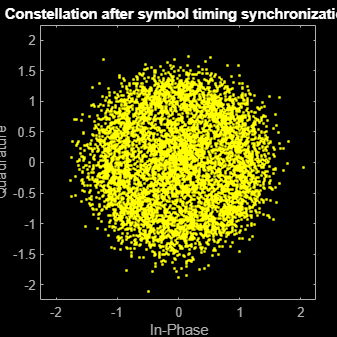```pause(0.5) % Error metrics display % For GS continuous streams if strcmpi(cfgDVBS2.StreamFormat,'GS') && ~rxParams.UPL if (simParams.numFrames-rxParams.totalSyncFrames == numFramesLost) fprintf("All frames are lost. No bits are retrieved from BB frames.") else ber = bitsErr/((dataStInd-rxParams.totalSyncFrames)*dataSize); fprintf('BER : %1.2e\n',ber) end else % For GS and TS packetized streams if pktsRec == 0 fprintf("All frames are lost. No packets are retrieved from BB frames.") else if strcmpi(cfgDVBS2.StreamFormat,'TS') pktLen = 1504; else pktLen = cfgDVBS2.UPL; % UP length including sync byte end ber = bitsErr/(pktsRec*pktLen); per = pktsErr/pktsRec; fprintf('PER: %1.2e\n',per) fprintf('BER: %1.2e\n',ber) end end```
```PER: 0.00e+00 ```
```BER: 0.00e+00 ```

### Further Exploration

The operating ${\mathit{E}}_{\mathit{s}}/{\mathit{N}}_{\mathit{o}}$ range of the DVB-S2 standard being very low requires the normalized loop bandwidth of the symbol synchronizer and coarse FLL to be very small for accurate estimation. These parameters are set via `rxParams.symbSyncLoopBW `and `rxParams.carrSyncLoopBW`.

#### Configure Symbol Timing Synchronization Parameters

Try running the simulation using the symbol timing synchronizer configured with a normalized loop bandwidth of 1e-4. With loop bandwidths at this level, this table shows the typical number of frames required for convergence of the symbol timing loop for specific modulation schemes and `'normal'` FEC frames.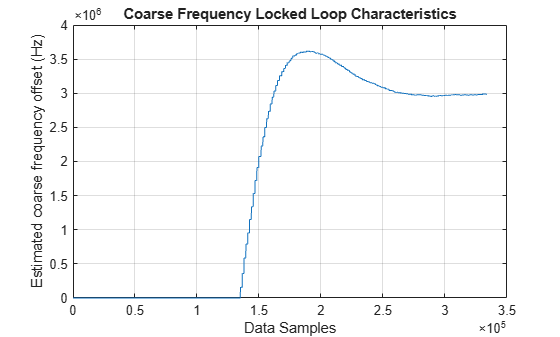For '`short`' FEC frames, the number of frames used for symbol timing synchronization is thrice the number required for '`normal`' FEC frames. To achieve convergence of the timing loop, the ratio `rxParams.symbSyncLoopBW/simParams.sps` must be greater than 1e-5. If the symbol timing loop doesn't converge, try increasing the `rxParams.carrSyncLoopBW` .

#### Configure Frame Synchronization Parameters

Choose a `rxParams.symbSyncLock` value from the table provided in Configure Symbol Timing Synchronization Parameters section. Set `rxParams.frameSyncLock` as a value in the range of [5, 15] frames based on the ${\mathit{E}}_{\mathit{s}}/{\mathit{N}}_{\mathit{o}}$ setting. If the output is not as expected, increase the number of frames required for frame synchronization.

#### Configure Carrier Synchronization Parameters

Try running the simulation using the coarse FLL configured with a normalized loop bandwidth of 1e-4*0.023 for PSK signals and 4e-4*0.023 for APSK signals.

When you set the `FECFrame` property to `'normal'`, set the `rxParams.coarseFreqLock` property to 20. When you set the `FECFrame` property to `'short'`, set the `rxParams.coarseFreqLock` property to 80. Set `simParams.EsNodB` to the lowest ${\mathit{E}}_{\mathit{s}}/{\mathit{N}}_{\mathit{o}}$ for the chosen modulation scheme from ETSI EN 302 307-1 Section 6 . For the `HelperDVBS2TimeFreqSynchronizer` system object, set its properties as discussed in the previous sections based on the chosen configuration.

```% timeFreqSync = HelperDVBS2TimeFreqSynchronizer( ... % 'CarrFreqLoopBW',rxParams.carrSyncLoopBW, ... % 'SymbTimeLoopBW',rxParams.symbSyncLoopBW, ... % 'SamplesPerSymbol',simParams.sps, ... % 'DataFrameSize',rxParams.xFecFrameSize, ... % 'SymbSyncTransitFrames',rxParams.symbSyncLock, ... % 'FrameSyncAveragingFrames',rxParams.frameSyncLock)```

Replace the code in the symbol timing and coarse frequency synchronization section with these lines of code. Run the simulation for different carrier frequency offset (CFO) values. After coarse frequency compensation, view the plot and the residual CFO value (`resCoarseCFO`) over each frame to observe the performance of the coarse frequency estimation algorithm. Ideally, the coarse frequency compensation reduces the error to 2% of the symbol rate. If the coarse frequency compensation is not reduced to less than 3% of the symbol rate, try decreasing the loop bandwidth and increasing the `rxParams.coarseFreqLock`. Because the frequency error is estimated using the pilot symbols, verify that the frame synchronizer is properly locked to the beginning of frame.

```% [out,index,phEst] = timeFreqSync(rxData,false); % Frequency offset estimate normalized by symbol rate % freqOffEst = diff(phEst(1:simParams.sps:end))/(2*pi); % plot(freqOffEst) % actFreqOff = simParams.cfo/(simParams.chanBW/(1 + cfgDVBS2.RolloffFactor)); % resCoarseCFO = abs(actFreqOff-freqOffEst(end));```

When the residual carrier frequency offset value (`resCoarseCFO`) is reduced to approximately 0.02 or 0.03, set the `rxParams.frameCount` to the `rxParams.coarseFreqLock` value.

For `'normal'` FEC frames, set the `rxParams.fineFreqLock` value to 10. For `'short'` FEC frames, set the `rxParams.fineFreqLock` value to 40. Replace the code in the fine frequency error estimation section with this code.

```% rxParams.fineFreqCorrVal = HelperDVBS2FineFreqEst(fineFreqIn(rxParams.pilotInd), ... % rxParams.numPilots,rxParams.refPilots,rxParams.fineFreqCorrVal); % fineFreqEst = angle(rxParams.fineFreqCorrVal)/(pi*(19)); % resFineCFO = abs(actFreqOff-freqOffEst(end)-fineFreqEst);```

Repeat the simulation process and observe the residual CFO value (`resFineCFO`) over each frame. If the fine frequency estimator does not reduce the residual carrier frequency error to approximately 0.01% of the symbol rate, try increasing the `rxParams.fineFreqLock` property value.

When the residual CFO value (`resFineCFO`) is reduced to approximately 0.0001 or 0.0002, set the `rxParams.frameCount+1` to the `rxParams.coarseFreqLock` value.

Fine phase compensation PLL is used for only 16 APSK and 32 APSK modulation schemes with substantial phase noise.

After refining the synchronization parameters set in the `rxParams` structure, perform the BER simulation for the updated configuration.

### Appendix

The example uses these helper functions:

### Bibliography

1. ETSI Standard EN 302 307-1 V1.4.1(2014-11). Digital Video Broadcasting (DVB); Second Generation Framing Structure, Channel Coding and Modulation Systems for Broadcasting, Interactive Services, News Gathering and other Broadband Satellite Applications (DVB-S2).

2. ETSI Standard TR 102 376-1 V1.2.1(2015-11). Digital Video Broadcasting (DVB); Implementation Guidelines for the Second Generation System for Broadcasting, Interactive Services, News Gathering and other Broadband Satellite Applications (DVB-S2).

3. Mengali, Umberto, and Aldo N.D'Andrea. Synchronization Techniques for Digital Receivers. New York: Plenum Press,1997.

4. E. Casini, R. De Gaudenzi, and Alberto Ginesi. "DVB‐S2 modem algorithms design and performance over typical satellite channels." International Journal of Satellite Communications and Networking 22, no. 3 (2004): 281-318.

5. Michael Rice, Digital Communications: A Discrete-Time Approach. New York: Prentice Hall, 2008.

## SupportGet trial now